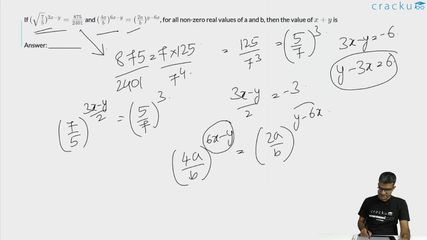Question 3

# If $$(\sqrt{\frac{7}{5}})^{3x-y}=\frac{875}{2401}$$ and $$(\frac{4a}{b})^{6x-y}=(\frac{2a}{b})^{y-6x}$$, for all non-zero real values of a and b, then the value of $$x+y$$ is

Solution

$$(\sqrt{\frac{7}{5}})^{3x-y}=\frac{875}{2401}$$

$$\left(\frac{7}{5}\right)^{\frac{\left(3x-y\right)}{2}}=\frac{125}{343}$$

$$\left(\frac{7}{5}\right)^{\frac{\left(3x-y\right)}{2}}=\left(\frac{7}{5}\right)^{-3}$$

3x-y = -6

$$(\frac{4a}{b})^{6x-y}=(\frac{2a}{b})^{y-6x}$$

Therefor, y=6x as the bases are different so the power should be zero for the results to be equal.

3x-y=-6

or, 3x - 6x = -6

or x= 2

y= 6x = 12

x+y = 14

### View Video Solution##### Anjali Chindalia

2 months, 1 week ago

Hello sir,
Is their any other method of solving this ques. As I didn't understood the solution

##### susheela rath

7 months, 1 week ago

Kutudu harami

##### susheela rath

7 months, 1 week ago

Dhhdhdgeue